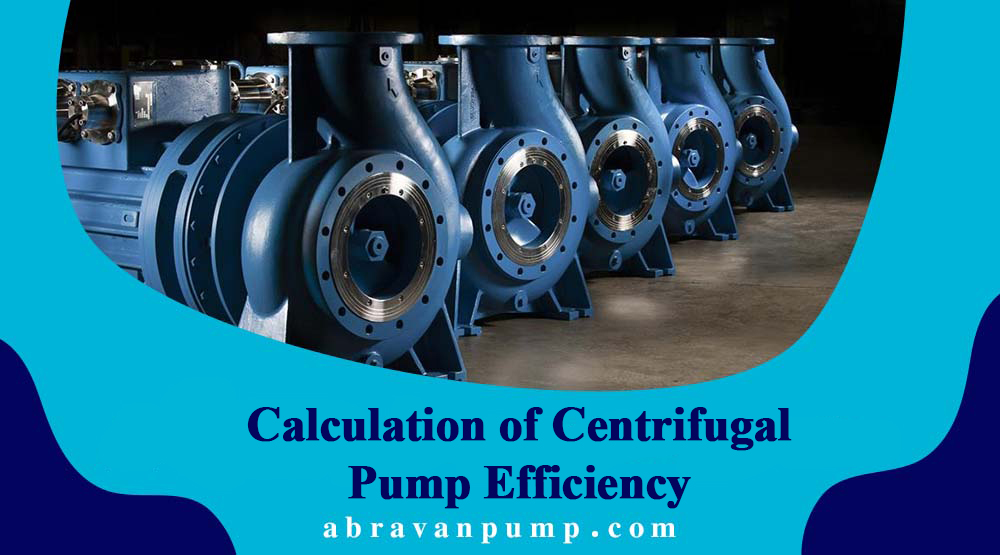صنایع پمپ سازی آبروان

# Calculation of Centrifugal Pump EfficiencyIn hydraulic machinery, as with other standard practices in fluid mechanics, prior to manufacturing a hydraulic machine for industrial needs, a model is constructed, adhering to the laws of similarity. Necessary experiments are conducted on the model, and the acquired results are applied to a prototype. To ensure geometric, kinematic, and dynamic similarity between the model and prototype (actual size), certain conditions must be met.

Kinematic similarity in centrifugal pumps is achieved by establishing similarity between velocity triangles at corresponding points on the impeller. To achieve dynamic similarity, it is imperative that the Reynolds numbers associated with fluid flow between the moving blades in both the model and prototype are equal. All similar machines with identical angles and constant dimensions will exhibit a characteristic H.

Hydraulic and volumetric efficiencies in model and prototype pumps are equal, but mechanical efficiency between the two undergoes slight changes. It should be noted that practically, hydraulic and volumetric efficiencies also experience changes due to the presence of different roughness coefficients between the model and prototype, as well as the existence of various seams between these two, which are attributed to the influence of scale. Without significant error, total efficiency, as well as hydraulic and mechanical efficiencies, can be considered equal between two similar pumps (model and prototype).

## Practical Application of the Laws of Similarity and Characteristic Curves of the Pumps

After manufacturing pumps, factories subject them to tests in their hydraulic laboratories to determine the created head by the pump (H), discharged flow rate (Q), absorbed power on the axis (N), and efficiency of the centrifugal pump (η). The characteristic performance of a centrifugal pump is resulted by plotting curves f1(Q)=H, N=f3(Q), and η=f2(Q) at a constant rotational speed, n=cte, as shown below.

N: Power absorbed on the pump axis

η: Overall pump efficiency

Q: Flow rate discharged by the pump

n: Rotational speed (r.p.m)

To plot characteristic curves of pumps, one can change the valve opening degree to adjust the water flow, altering the discharge flow rate Q by the pump. Consequently, the created head H, absorbed power N, and efficiency η corresponding to each flow rate can be obtained at a constant rotational speed, and the results can be graphically represented.

The pump should operate in a regime where the pump efficiency is maximum. Typically, an appropriate pump efficiency for the pump operating regime is chosen around 95-93%, close to the maximum pump efficiency. By using characteristic curves of pump performance, it becomes straightforward to select the desired pump suitable for working conditions.

Using the characteristic curves of a pump, the following issues can be addressed:

1. Determining the required rotational speed n to achieve the flow rate Q and head H.
2. Determining the head created by the pump, H, with known rotational speed n and discharged flow rate Q.
3. Determining the flow rate Q by the pump with known rotational speed n and head created by the pump, H.
4. Power on the pump axis can be obtained by measuring the rotational speed on the pump axis and coupling.

Abravan Company offers centrifugal pumps among its products. For purchasing a centrifugal pump, you can contact the company’s experts for guidance.

+982166938814
+982166435453
+982166924294
+982166573503-4

abravanpump.com/?p=36255

Abravan Pump

پـمـپ سـازی آبـروان